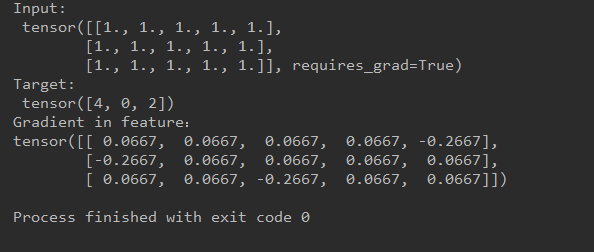# Why the gradient of feature passing into CrossEntroyLoss function is different from the theoretical value？

Here is my code, it’s similar to official example.

``````import math
import torch
import torch.nn as nn
import torch.nn.functional as F

loss = nn.CrossEntropyLoss()
target = torch.empty(3, dtype=torch.long).random_(5)

output_sm = F.log_softmax(feature, dim=1)
output_nll = F.nll_loss(output_sm, target)
output = output_nll
output.backward()

print("Input:\n", feature)
print("Target:\n", target)
``````

The picture below is my results.The result of theoretical deduction is as follow:

Partial derivative of L to z equals p - y

L represents loss function
z is the input feature
p is the output of softmax
y is the target
Regarding the detailed derivation of this formula, I am not here because it is easy to get.

What confused me is the experimental results do not match the theoretical values?
Can you give me some suggestion? Thank you!

What is the value you expect?
Given there is a log then softmax then nll then averaging. Plus the backward computations of each. I wouldn’t say it’s a trivial computation to do.

This is the formula.p = [
0.2 0.2  0.2 0.2 -0.8
-0.8 0.2  0.2 0.2 0.2
0.2 0.2 -0.8 0.2 0.2
]

y = [4, 0, 2]

The values I expected as follows:

0.2 0.2  0.2 0.2 -0.8
-0.8 0.2  0.2 0.2 0.2
0.2 0.2 -0.8 0.2 0.2
]

Could you explain how you get `dL/dz = p - y` please?

Thank you! I have solved this problem. I did not divide by batches when caculating.
I will explain how I get `dL/dz = p - y` later.

1 Like